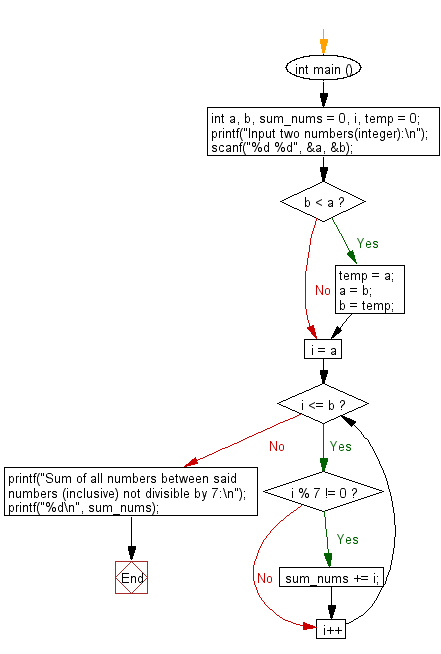﻿ C : Add all numbers not divisible by 7 between two numbers

# C Exercises: Sum all numbers between two given numbers (inclusive) not divisible by seven

## C Basic Declarations and Expressions: Exercise-119 with Solution

Write a C program to calculate the sum of all numbers between two given numbers (inclusive) not divisible by 7.

Sample Solution:

C Code:

``````#include <stdio.h>

int main () {
int a, b, sum_nums = 0, i, temp = 0;

// Prompt user for input
printf("Input two numbers(integer):\n");

// Read two integer values from user and store them in 'a' and 'b'
scanf("%d %d", &a, &b);

// Swap 'a' and 'b' if 'b' is smaller than 'a'
if (b < a) {
temp = a;
a = b;
b = temp;
}

// Loop through the range from 'a' to 'b' (inclusive)
for (i = a; i <= b; i++) {
// Check if 'i' is not divisible by 7
if (i % 7 != 0) {
sum_nums += i; // Accumulate numbers not divisible by 7
}
}

// Print the sum of numbers not divisible by 7
printf("Sum of all numbers between said numbers (inclusive) not divisible by 7:\n");
printf("%d\n", sum_nums);

return 0; // End of program
}
``````

Sample Output:

```Input two numbers(integer):
25
5
Sum of all numbers between said numbers (inclusive) not divisible by 7:
273
```

Sample Output:

```Input two numbers(integer):
6
36
Sum of all numbers between said numbers (inclusive) not divisible by 7:
546
```

Flowchart:C programming Code Editor:

What is the difficulty level of this exercise?

Test your Programming skills with w3resource's quiz.

﻿